Search by Topic

Resources tagged with Generalising similar to Ladybirds in the Garden:

Filter by: Content type:
Age range:
Challenge level:

There are 90 results

Broad Topics > Using, Applying and Reasoning about Mathematics > Generalising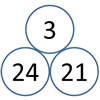Unit Differences

Age 5 to 7 Challenge Level:

This challenge is about finding the difference between numbers which have the same tens digit.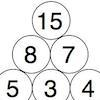Build it up More

Age 7 to 11 Challenge Level:

This task follows on from Build it Up and takes the ideas into three dimensions!Sums and Differences 1

Age 7 to 11 Challenge Level:

This challenge focuses on finding the sum and difference of pairs of two-digit numbers.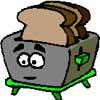Broken Toaster

Age 7 to 11 Short Challenge Level:

Only one side of a two-slice toaster is working. What is the quickest way to toast both sides of three slices of bread?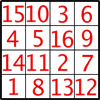Magic Constants

Age 7 to 11 Challenge Level:

In a Magic Square all the rows, columns and diagonals add to the 'Magic Constant'. How would you change the magic constant of this square?Sums and Differences 2

Age 7 to 11 Challenge Level:

Find the sum and difference between a pair of two-digit numbers. Now find the sum and difference between the sum and difference! What happens?Lots of Lollies

Age 5 to 7 Challenge Level:

Frances and Rishi were given a bag of lollies. They shared them out evenly and had one left over. How many lollies could there have been in the bag?Button-up Some More

Age 7 to 11 Challenge Level:

How many ways can you find to do up all four buttons on my coat? How about if I had five buttons? Six ...?Build it Up

Age 7 to 11 Challenge Level:

Can you find all the ways to get 15 at the top of this triangle of numbers? Many opportunities to work in different ways.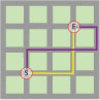The Add and Take-away Path

Age 5 to 7 Challenge Level:

Two children made up a game as they walked along the garden paths. Can you find out their scores? Can you find some paths of your own?Dice Stairs

Age 7 to 11 Challenge Level:

Can you make dice stairs using the rules stated? How do you know you have all the possible stairs?Division Rules

Age 7 to 11 Challenge Level:

This challenge encourages you to explore dividing a three-digit number by a single-digit number.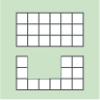Area and Perimeter

Age 7 to 11 Challenge Level:

What can you say about these shapes? This problem challenges you to create shapes with different areas and perimeters.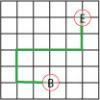Journeys in Numberland

Age 7 to 11 Challenge Level:

Tom and Ben visited Numberland. Use the maps to work out the number of points each of their routes scores.Sitting Round the Party Tables

Age 5 to 11 Challenge Level:

Sweets are given out to party-goers in a particular way. Investigate the total number of sweets received by people sitting in different positions.Centred Squares

Age 7 to 11 Challenge Level:

This challenge, written for the Young Mathematicians' Award, invites you to explore 'centred squares'.Doplication

Age 7 to 11 Challenge Level:

We can arrange dots in a similar way to the 5 on a dice and they usually sit quite well into a rectangular shape. How many altogether in this 3 by 5? What happens for other sizes?Growing Garlic

Age 5 to 7 Challenge Level:

Ben and his mum are planting garlic. Use the interactivity to help you find out how many cloves of garlic they might have had.Tiling

Age 7 to 11 Challenge Level:

An investigation that gives you the opportunity to make and justify predictions.Bundles of Cubes

Age 7 to 11 Challenge Level:

Watch this animation. What do you notice? What happens when you try more or fewer cubes in a bundle?Calendar Calculations

Age 7 to 11 Challenge Level:

Try adding together the dates of all the days in one week. Now multiply the first date by 7 and add 21. Can you explain what happens?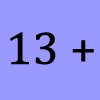What Could it Be?

Age 5 to 7 Challenge Level:

In this calculation, the box represents a missing digit. What could the digit be? What would the solution be in each case?Sticky Triangles

Age 7 to 11 Challenge Level:

Can you continue this pattern of triangles and begin to predict how many sticks are used for each new "layer"?Cut it Out

Age 7 to 11 Challenge Level:

Can you dissect an equilateral triangle into 6 smaller ones? What number of smaller equilateral triangles is it NOT possible to dissect a larger equilateral triangle into?Simple Train Journeys

Age 5 to 11 Challenge Level:

How many different journeys could you make if you were going to visit four stations in this network? How about if there were five stations? Can you predict the number of journeys for seven stations?Number Differences

Age 7 to 11 Challenge Level:

Place the numbers from 1 to 9 in the squares below so that the difference between joined squares is odd. How many different ways can you do this?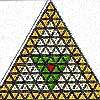The Great Tiling Count

Age 7 to 11 Challenge Level:

Compare the numbers of particular tiles in one or all of these three designs, inspired by the floor tiles of a church in Cambridge.Round the Two Dice

Age 5 to 7 Challenge Level:

This activity focuses on rounding to the nearest 10.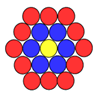Counting Counters

Age 7 to 11 Challenge Level:

Take a counter and surround it by a ring of other counters that MUST touch two others. How many are needed?Oddly

Age 7 to 11 Challenge Level:

Find the sum of all three-digit numbers each of whose digits is odd.Round the Three Dice

Age 7 to 11 Challenge Level:

What happens when you round these three-digit numbers to the nearest 100?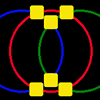Magic Circles

Age 7 to 11 Challenge Level:

Put the numbers 1, 2, 3, 4, 5, 6 into the squares so that the numbers on each circle add up to the same amount. Can you find the rule for giving another set of six numbers?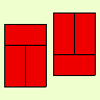Fault-free Rectangles

Age 7 to 11 Challenge Level:

Find out what a "fault-free" rectangle is and try to make some of your own.Move a Match

Age 7 to 11 Challenge Level:

How can you arrange these 10 matches in four piles so that when you move one match from three of the piles into the fourth, you end up with the same arrangement?Age 5 to 11 Challenge Level:

Try out this number trick. What happens with different starting numbers? What do you notice?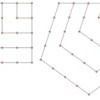Polygonals

Age 7 to 11 Challenge Level:

Polygonal numbers are those that are arranged in shapes as they enlarge. Explore the polygonal numbers drawn here.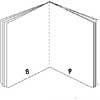Lost Books

Age 7 to 11 Challenge Level:

While we were sorting some papers we found 3 strange sheets which seemed to come from small books but there were page numbers at the foot of each page. Did the pages come from the same book?Domino Numbers

Age 7 to 11 Challenge Level:

Can you see why 2 by 2 could be 5? Can you predict what 2 by 10 will be?How Odd

Age 5 to 7 Challenge Level:

This problem challenges you to find out how many odd numbers there are between pairs of numbers. Can you find a pair of numbers that has four odds between them?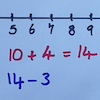Strike it Out

Age 5 to 11 Challenge Level:

Use your addition and subtraction skills, combined with some strategic thinking, to beat your partner at this game.Surprising Split

Age 7 to 11 Challenge Level:

Does this 'trick' for calculating multiples of 11 always work? Why or why not?Round the Dice Decimals 1

Age 7 to 11 Challenge Level:

Use two dice to generate two numbers with one decimal place. What happens when you round these numbers to the nearest whole number?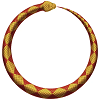Snake Coils

Age 7 to 11 Challenge Level:

This challenge asks you to imagine a snake coiling on itself.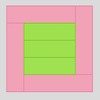Cuisenaire Rods

Age 7 to 11 Challenge Level:

These squares have been made from Cuisenaire rods. Can you describe the pattern? What would the next square look like?Round the Dice Decimals 2

Age 7 to 11 Challenge Level:

What happens when you round these numbers to the nearest whole number?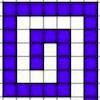Spirals, Spirals

Age 7 to 11 Challenge Level:

Here are two kinds of spirals for you to explore. What do you notice?Circles, Circles

Age 5 to 11 Challenge Level:

Here are some arrangements of circles. How many circles would I need to make the next size up for each? Can you create your own arrangement and investigate the number of circles it needs?Round and Round the Circle

Age 7 to 11 Challenge Level:

What happens if you join every second point on this circle? How about every third point? Try with different steps and see if you can predict what will happen.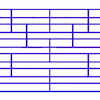Tumbling Down

Age 7 to 11 Challenge Level:

Watch this animation. What do you see? Can you explain why this happens?Window Frames

Age 5 to 14 Challenge Level:

This task encourages you to investigate the number of edging pieces and panes in different sized windows.